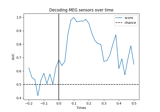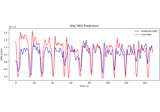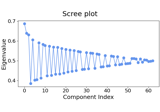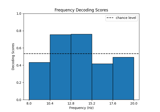# mne.decoding.CSP¶

class mne.decoding.CSP(n_components=4, reg=None, log=None, cov_est='concat', transform_into='average_power', norm_trace=False, cov_method_params=None, rank=None, component_order='mutual_info')[source]

M/EEG signal decomposition using the Common Spatial Patterns (CSP).

This class can be used as a supervised decomposition to estimate spatial filters for feature extraction. CSP in the context of EEG was first described in 1; a comprehensive tutorial on CSP can be found in 2. Multi-class solving is implemented from 3.

Parameters
n_components`int` (default 4)

The number of components to decompose M/EEG signals. This number should be set by cross-validation.

reg

If not None (same as `'empirical'`, default), allow regularization for covariance estimation. If float (between 0 and 1), shrinkage is used. For str values, `reg` will be passed as `method` to `mne.compute_covariance()`.

log`None` | bool (default `None`)

If `transform_into` equals `'average_power'` and `log` is None or True, then apply a log transform to standardize features, else features are z-scored. If `transform_into` is `'csp_space'`, `log` must be None.

cov_est‘concat’ | ‘epoch’ (default ‘concat’)

If `'concat'`, covariance matrices are estimated on concatenated epochs for each class. If `'epoch'`, covariance matrices are estimated on each epoch separately and then averaged over each class.

transform_into‘average_power’ | ‘csp_space’ (default ‘average_power’)

If ‘average_power’ then `self.transform` will return the average power of each spatial filter. If `'csp_space'`, `self.transform` will return the data in CSP space.

norm_tracebool (default `False`)

Normalize class covariance by its trace. Trace normalization is a step of the original CSP algorithm 1 to eliminate magnitude variations in the EEG between individuals. It is not applied in more recent work 2, 3 and can have a negative impact on pattern order.

cov_method_params

Parameters to pass to `mne.compute_covariance()`.

New in version 0.16.

rank`None` | ‘info’ | ‘full’ | `dict`

This controls the rank computation that can be read from the measurement info or estimated from the data. When a noise covariance is used for whitening, this should reflect the rank of that covariance, otherwise amplification of noise components can occur in whitening (e.g., often during source localization).

`None`

The rank will be estimated from the data after proper scaling of different channel types.

`'info'`

The rank is inferred from `info`. If data have been processed with Maxwell filtering, the Maxwell filtering header is used. Otherwise, the channel counts themselves are used. In both cases, the number of projectors is subtracted from the (effective) number of channels in the data. For example, if Maxwell filtering reduces the rank to 68, with two projectors the returned value will be 66.

`'full'`

The rank is assumed to be full, i.e. equal to the number of good channels. If a `Covariance` is passed, this can make sense if it has been (possibly improperly) regularized without taking into account the true data rank.

`dict`

Calculate the rank only for a subset of channel types, and explicitly specify the rank for the remaining channel types. This can be extremely useful if you already know the rank of (part of) your data, for instance in case you have calculated it earlier.

This parameter must be a dictionary whose keys correspond to channel types in the data (e.g. `'meg'`, `'mag'`, `'grad'`, `'eeg'`), and whose values are integers representing the respective ranks. For example, `{'mag': 90, 'eeg': 45}` will assume a rank of `90` and `45` for magnetometer data and EEG data, respectively.

The ranks for all channel types present in the data, but not specified in the dictionary will be estimated empirically. That is, if you passed a dataset containing magnetometer, gradiometer, and EEG data together with the dictionary from the previous example, only the gradiometer rank would be determined, while the specified magnetometer and EEG ranks would be taken for granted.

The default is `None`.

New in version 0.17.

component_order‘mutual_info’ | ‘alternate’ (default ‘mutual_info’)

If `'mutual_info'` order components by decreasing mutual information (in the two-class case this uses a simplification which orders components by decreasing absolute deviation of the eigenvalues from 0.5 4). For the two-class case, `'alternate'` orders components by starting with the largest eigenvalue, followed by the smallest, the second-to-largest, the second-to-smallest, and so on 2.

New in version 0.21.

References

1(1,2)

Zoltan J. Koles, Michael S. Lazar, and Steven Z. Zhou. Spatial patterns underlying population differences in the background EEG. Brain Topography, 2(4):275–284, 1990. doi:10.1007/BF01129656.

2(1,2,3)

Benjamin Blankertz, Ryota Tomioka, Steven Lemm, Motoaki Kawanabe, and Klaus-Robert Müller. Optimizing spatial filters for robust EEG single-trial analysis. IEEE Signal Processing Magazine, 25(1):41–56, 2008. doi:10.1109/MSP.2008.4408441.

3(1,2)

Moritz Grosse-Wentrup and Martin Buss. Multiclass common spatial patterns and information theoretic feature extraction. IEEE Transactions on Biomedical Engineering, 55(8):1991–2000, 2008. doi:10.1109/TBME.2008.921154.

4

Alexandre Barachant, Stephane Bonnet, Marco Congedo, and Christian Jutten. Common spatial pattern revisited by Riemannian geometry. In 2010 IEEE International Workshop on Multimedia Signal Processing, 472–476. 2010. doi:10.1109/MMSP.2010.5662067.

Attributes
filters_`ndarray`, shape (n_channels, n_channels)

If fit, the CSP components used to decompose the data, else None.

patterns_`ndarray`, shape (n_channels, n_channels)

If fit, the CSP patterns used to restore M/EEG signals, else None.

mean_`ndarray`, shape (n_components,)

If fit, the mean squared power for each component.

std_`ndarray`, shape (n_components,)

If fit, the std squared power for each component.

Methods

 `__hash__`(/) Return hash(self). `fit`(X, y) Estimate the CSP decomposition on epochs. `fit_transform`(X[, y]) Fit to data, then transform it. `get_params`([deep]) Get parameters for this estimator. `plot_filters`(info[, components, ch_type, ...]) Plot topographic filters of components. `plot_patterns`(info[, components, ch_type, ...]) Plot topographic patterns of components. `set_params`(**params) Set the parameters of this estimator. Estimate epochs sources given the CSP filters.
fit(X, y)[source]

Estimate the CSP decomposition on epochs.

Parameters
X`ndarray`, shape (n_epochs, n_channels, n_times)

The data on which to estimate the CSP.

y`array`, shape (n_epochs,)

The class for each epoch.

Returns
selfinstance of `CSP`

Returns the modified instance.

Examples using `fit`:fit_transform(X, y=None, **fit_params)[source]

Fit to data, then transform it.

Fits transformer to X and y with optional parameters fit_params and returns a transformed version of X.

Parameters
X`array`, shape (n_samples, n_features)

Training set.

y`array`, shape (n_samples,)

Target values.

**fit_params`dict`

Additional fitting parameters passed to `self.fit`.

Returns
X_new`array`, shape (n_samples, n_features_new)

Transformed array.

Examples using `fit_transform`:get_params(deep=True)[source]

Get parameters for this estimator.

Parameters
deepbool, optional

If True, will return the parameters for this estimator and contained subobjects that are estimators.

Returns
params`dict`

Parameter names mapped to their values.

plot_filters(info, components=None, ch_type=None, vmin=None, vmax=None, cmap='RdBu_r', sensors=True, colorbar=True, scalings=None, units='a.u.', res=64, size=1, cbar_fmt='%3.1f', name_format='CSP%01d', show=True, show_names=False, title=None, mask=None, mask_params=None, outlines='head', contours=6, image_interp='bilinear', average=None)[source]

Plot topographic filters of components.

The filters are used to extract discriminant neural sources from the measured data (a.k.a. the backward model).

Parameters
info`mne.Info`

The `mne.Info` object with information about the sensors and methods of measurement. Used for fitting. If not available, consider using `mne.create_info()`.

components

The patterns to plot. If None, n_components will be shown.

ch_type‘mag’ | ‘grad’ | ‘planar1’ | ‘planar2’ | ‘eeg’ | `None`

The channel type to plot. For ‘grad’, the gradiometers are collected in pairs and the RMS for each pair is plotted. If None, then first available channel type from order given above is used. Defaults to None.

vmin

The value specifying the lower bound of the color range. If None, and vmax is None, -vmax is used. Else np.min(data). If callable, the output equals vmin(data).

vmax

The value specifying the upper bound of the color range. If None, the maximum absolute value is used. If vmin is None, but vmax is not, defaults to np.min(data). If callable, the output equals vmax(data).

cmapmatplotlib colormap | (colormap, bool) | ‘interactive’ | `None`

Colormap to use. If tuple, the first value indicates the colormap to use and the second value is a boolean defining interactivity. In interactive mode the colors are adjustable by clicking and dragging the colorbar with left and right mouse button. Left mouse button moves the scale up and down and right mouse button adjusts the range. Hitting space bar resets the range. Up and down arrows can be used to change the colormap. If None, ‘Reds’ is used for all positive data, otherwise defaults to ‘RdBu_r’. If ‘interactive’, translates to (None, True). Defaults to ‘RdBu_r’.

Warning

Interactive mode works smoothly only for a small amount of topomaps.

sensors

Add markers for sensor locations to the plot. Accepts matplotlib plot format string (e.g., ‘r+’ for red plusses). If True, a circle will be used (via .add_artist). Defaults to True.

colorbarbool

Plot a colorbar.

scalings

The scalings of the channel types to be applied for plotting. If None, defaults to `dict(eeg=1e6, grad=1e13, mag=1e15)`.

units

The unit of the channel type used for colorbar label. If scale is None the unit is automatically determined.

res`int`

The resolution of the topomap image (n pixels along each side).

size`float`

Side length per topomap in inches.

cbar_fmt`str`

String format for colorbar values.

name_format`str`

String format for topomap values. Defaults to “CSP%01d”.

showbool

Show figure if True.

show_names

If True, show channel names on top of the map. If a callable is passed, channel names will be formatted using the callable; e.g., to delete the prefix ‘MEG ‘ from all channel names, pass the function lambda x: x.replace(‘MEG ‘, ‘’). If `mask` is not None, only significant sensors will be shown.

title

Title. If None (default), no title is displayed.

mask`ndarray` of bool, shape (n_channels, n_times) | `None`

The channels to be marked as significant at a given time point. Indices set to `True` will be considered. Defaults to None.

Additional plotting parameters for plotting significant sensors. Default (None) equals:

```dict(marker='o', markerfacecolor='w', markeredgecolor='k',
linewidth=0, markersize=4)
```
outlines‘head’ | ‘skirt’ | `dict` | `None`

The outlines to be drawn. If ‘head’, the default head scheme will be drawn. If ‘skirt’ the head scheme will be drawn, but sensors are allowed to be plotted outside of the head circle. If dict, each key refers to a tuple of x and y positions, the values in ‘mask_pos’ will serve as image mask. Alternatively, a matplotlib patch object can be passed for advanced masking options, either directly or as a function that returns patches (required for multi-axis plots). If None, nothing will be drawn. Defaults to ‘head’.

contours

The number of contour lines to draw. If 0, no contours will be drawn. When an integer, matplotlib ticker locator is used to find suitable values for the contour thresholds (may sometimes be inaccurate, use array for accuracy). If an array, the values represent the levels for the contours. Defaults to 6.

image_interp`str`

The image interpolation to be used. All matplotlib options are accepted.

average

The time window around a given time to be used for averaging (seconds). For example, 0.01 would translate into window that starts 5 ms before and ends 5 ms after a given time point. Defaults to None, which means no averaging.

Returns
figinstance of `matplotlib.figure.Figure`

The figure.

Examples using `plot_filters`:plot_patterns(info, components=None, ch_type=None, vmin=None, vmax=None, cmap='RdBu_r', sensors=True, colorbar=True, scalings=None, units='a.u.', res=64, size=1, cbar_fmt='%3.1f', name_format='CSP%01d', show=True, show_names=False, title=None, mask=None, mask_params=None, outlines='head', contours=6, image_interp='bilinear', average=None, sphere=None)[source]

Plot topographic patterns of components.

The patterns explain how the measured data was generated from the neural sources (a.k.a. the forward model).

Parameters
info`mne.Info`

The `mne.Info` object with information about the sensors and methods of measurement. Used for fitting. If not available, consider using `mne.create_info()`.

components

The patterns to plot. If None, n_components will be shown.

ch_type‘mag’ | ‘grad’ | ‘planar1’ | ‘planar2’ | ‘eeg’ | `None`

The channel type to plot. For ‘grad’, the gradiometers are collected in pairs and the RMS for each pair is plotted. If None, then first available channel type from order given above is used. Defaults to None.

vmin

The value specifying the lower bound of the color range. If None, and vmax is None, -vmax is used. Else np.min(data). If callable, the output equals vmin(data).

vmax

The value specifying the upper bound of the color range. If None, the maximum absolute value is used. If vmin is None, but vmax is not, default np.min(data). If callable, the output equals vmax(data).

cmapmatplotlib colormap | (colormap, bool) | ‘interactive’ | `None`

Colormap to use. If tuple, the first value indicates the colormap to use and the second value is a boolean defining interactivity. In interactive mode the colors are adjustable by clicking and dragging the colorbar with left and right mouse button. Left mouse button moves the scale up and down and right mouse button adjusts the range. Hitting space bar resets the range. Up and down arrows can be used to change the colormap. If None, ‘Reds’ is used for all positive data, otherwise defaults to ‘RdBu_r’. If ‘interactive’, translates to (None, True). Defaults to ‘RdBu_r’.

Warning

Interactive mode works smoothly only for a small amount of topomaps.

sensors

Add markers for sensor locations to the plot. Accepts matplotlib plot format string (e.g., ‘r+’ for red plusses). If True, a circle will be used (via .add_artist). Defaults to True.

colorbarbool

Plot a colorbar.

scalings

The scalings of the channel types to be applied for plotting. If None, defaults to `dict(eeg=1e6, grad=1e13, mag=1e15)`.

units

The unit of the channel type used for colorbar label. If scale is None the unit is automatically determined.

res`int`

The resolution of the topomap image (n pixels along each side).

size`float`

Side length per topomap in inches.

cbar_fmt`str`

String format for colorbar values.

name_format`str`

String format for topomap values. Defaults to “CSP%01d”.

showbool

Show figure if True.

show_names

If True, show channel names on top of the map. If a callable is passed, channel names will be formatted using the callable; e.g., to delete the prefix ‘MEG ‘ from all channel names, pass the function lambda x: x.replace(‘MEG ‘, ‘’). If `mask` is not None, only significant sensors will be shown.

title

Title. If None (default), no title is displayed.

mask`ndarray` of bool, shape (n_channels, n_patterns) | `None`

Array indicating channel-pattern combinations to highlight with a distinct plotting style. Array elements set to `True` will be plotted with the parameters given in `mask_params`. Defaults to `None`, equivalent to an array of all `False` elements.

Additional plotting parameters for plotting significant sensors. Default (None) equals:

```dict(marker='o', markerfacecolor='w', markeredgecolor='k',
linewidth=0, markersize=4)
```
outlines‘head’ | ‘skirt’ | `dict` | `None`

The outlines to be drawn. If ‘head’, the default head scheme will be drawn. If ‘skirt’ the head scheme will be drawn, but sensors are allowed to be plotted outside of the head circle. If dict, each key refers to a tuple of x and y positions, the values in ‘mask_pos’ will serve as image mask. Alternatively, a matplotlib patch object can be passed for advanced masking options, either directly or as a function that returns patches (required for multi-axis plots). If None, nothing will be drawn. Defaults to ‘head’.

contours

The number of contour lines to draw. If 0, no contours will be drawn. When an integer, matplotlib ticker locator is used to find suitable values for the contour thresholds (may sometimes be inaccurate, use array for accuracy). If an array, the values represent the levels for the contours. Defaults to 6.

image_interp`str`

The image interpolation to be used. All matplotlib options are accepted.

average

The time window around a given time to be used for averaging (seconds). For example, 0.01 would translate into window that starts 5 ms before and ends 5 ms after a given time point. Defaults to None, which means no averaging.

sphere

The sphere parameters to use for the cartoon head. Can be array-like of shape (4,) to give the X/Y/Z origin and radius in meters, or a single float to give the radius (origin assumed 0, 0, 0). Can also be a spherical ConductorModel, which will use the origin and radius. Can be “auto” to use a digitization-based fit. Can also be None (default) to use ‘auto’ when enough extra digitization points are available, and 0.095 otherwise. Currently the head radius does not affect plotting.

New in version 0.20.

Returns
figinstance of `matplotlib.figure.Figure`

The figure.

Examples using `plot_patterns`:set_params(**params)[source]

Set the parameters of this estimator.

The method works on simple estimators as well as on nested objects (such as pipelines). The latter have parameters of the form `<component>__<parameter>` so that it’s possible to update each component of a nested object.

Parameters
**params`dict`

Parameters.

Returns
instinstance

The object.

transform(X)[source]

Estimate epochs sources given the CSP filters.

Parameters
X`array`, shape (n_epochs, n_channels, n_times)

The data.

Returns
X`ndarray`

If self.transform_into == ‘average_power’ then returns the power of CSP features averaged over time and shape (n_epochs, n_sources) If self.transform_into == ‘csp_space’ then returns the data in CSP space and shape is (n_epochs, n_sources, n_times).

Examples using `transform`:## Examples using `mne.decoding.CSP`¶